﻿ Managing the Uncertainty in the van Hiele Levels of Geometric Reasoning

### Managing the Uncertainty in the van Hiele Levels of Geometric Reasoning

Michael Gr. Voskoglou

American Journal of Educational Research

## Managing the Uncertainty in the van Hiele Levels of Geometric Reasoning

Michael Gr. VoskoglouMathematical Sciences, School of Technological Applications, Graduate Technological Educational Institute of Western Greece, Patras, Greece

### Abstract

Student group total possibilistic uncertainty and probabilistic uncertainty are calculated for evaluating the student acquisition of the van Hiele levels of geometric reasoning and an example is presented illustrating our results.

• Michael Gr. Voskoglou. Managing the Uncertainty in the van Hiele Levels of Geometric Reasoning. American Journal of Educational Research. Vol. 5, No. 2, 2017, pp 109-113. http://pubs.sciepub.com/education/5/2/1
• Voskoglou, Michael Gr.. "Managing the Uncertainty in the van Hiele Levels of Geometric Reasoning." American Journal of Educational Research 5.2 (2017): 109-113.
• Voskoglou, M. G. (2017). Managing the Uncertainty in the van Hiele Levels of Geometric Reasoning. American Journal of Educational Research, 5(2), 109-113.
• Voskoglou, Michael Gr.. "Managing the Uncertainty in the van Hiele Levels of Geometric Reasoning." American Journal of Educational Research 5, no. 2 (2017): 109-113.

 Import into BibTeX Import into EndNote Import into RefMan Import into RefWorks

### 1. Introduction

Situations appear frequently in our day to day life characterized by a degree of uncertainty and/or fuzziness. In Education for example, a teacher is frequently not sure about the degree of acquisition of the new knowledge by students, or for the proper mark to characterize a student’s performance. There used to be a tradition in science and engineering of turning to probability theory when a problem was faced in which uncertainty played an important role. Today this is no longer the rule. Fuzzy Logic (FL), due to its property of characterizing the ambiguous cases with multiple values, provides a rich and meaningful addition to standard logic enabling the mathematical modeling under conditions which are imprecisely defined, despite the concerns of classical logicians.

This article’s author, starting in 1999 with a model for the process of learning a subject matter in the classroom , has frequently utilized principles of FL in past for describing and evaluating several human or machine (e.g. Case-Based Reasoning systems in computers ) activities. In particular, the measurement of a fuzzy system’s probabilistic or possibilistic uncertainty is one of the methods used in several earlier works, e.g. [16, 17, 18, 21], etc.

In the present article the corresponding fuzzy system’s uncertainty is used to evaluate the acquisition by students of the van Hiele (vH) levels of geometric reasoning. The rest of the paper is formulated as follows: In Section 2 we give a brief account of the vH level theory and of several previous researches on it connected to the present work. In Section 3 we deal with the main types of a fuzzy system’s uncertainty, while in Section 4 we give an example illustrating their applicability to evaluate the acquisition of the vH levels in Geometry by high school students. We close with Section 5 including our conclusion and some hints for future research.

For general facts on Fuzzy Sets (FS) and the uncertainty connected to them we refer to the book . We recall here that a FS on the universal set of the discourse U (or a fuzzy subset of U) is a set of ordered pairs of the form A = {(x, m(x)): x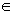U}, where m: U[0, 1] is its membership function. The closer is the membership degree y=m(x) of x to 1, the better x satisfies the characteristic property of A.

Many authors identify for reasons of simplicity a fuzzy set with its membership function, the choice of which is not unique, depending upon the user’s personal criteria and goals. However, in order to represent correctly the corresponding real situation, the choice of the membership functions must be compatible to the common logic.

### 2. The van Hiele Levels of Geometric Reasoning

The pedagogical value of the Euclidian geometry is great, mainly because it cultivates the student cognitive skills and connects directly mathematics to the real world. However, students face many difficulties for the learning of the Euclidian geometry, which fluctuate from the understanding of the space to the development of geometric reasoning and the ability of constructing the proofs and solutions of several geometric propositions and problems.

The vH theory of geometric reasoning [5, 6, 13, 14] suggests that students can progress through five levels of increasing structural complexity. A higher level contains all knowledge of any lower level and some additional knowledge which is not explicit at the lower levels. Therefore, each level appears as a meta-theory of the previous one . The five vH levels include:

• L1 (Visualization): Students perceive the geometric figures as entities according to their appearance, without explicit regard to their properties.

• L2 (Analysis): Students establish the properties of geometric figures by means of an informal analysis of their component parts and begin to recognize them by their properties.

• L3 (Abstraction): Students become able to relate the properties of figures, to distinguish between the necessity and sufficiency of a set of properties in determining a concept and to form abstract definitions.

• L4 (Deduction): Students reason formally within the context of a geometric system and they gasp the significance of deduction as means of developing geometric theory.

• L5 (Rigor): Students understand the foundations of geometry and can compare geometric systems based on different axioms.

Obviously the level L5 is very difficult, if not impossible, to appear in secondary classrooms, whereas level L4 also appears very rarely.

Although van Hiele  claimed that the above levels are discrete – which means that the transition from a level to the next one does not happen gradually but all at once – alternative researches by Burnes & Shaughnessy , Fuys et al. , Wilson , Guttierrez et al. , and by Perdikaris  suggest that the vH levels are continuous characterized by transitions between the adjacent levels. This means that from the teacher’s point of view there exists fuzziness about the degree of student acquisition of each vH level. Therefore, principles of FL can be used for the assessment of student geometric reasoning skills.

### 3. Types of Uncertainty in Fuzzy Systems

Uncertainty is the shortage of precise knowledge and of complete information on data, which describe together the state of the corresponding system. One of the key problems of artificial intelligence is the modelling of the uncertainty for solving real life problems and several models have been proposed for this purpose.

The amount of information obtained by an action can be measured by the reduction of the uncertainty resulting from this action. Therefore, a system’s uncertainty is connected to its capacity for obtaining relevant information. Accordingly a measure of uncertainty could be adopted as a measure of a system’s effectiveness in solving related problems. The greater is the decrease of the uncertainty resulting from the action (i.e. the difference of the existing uncertainty before and after the action), the better the system’s performance with respect to the action.

In terms of the classical probability theory a system’s uncertainty and the information connected to it are measured by the Shannon’s formula, better known as the Shannon’s entropy{1} . For use in a fuzzy environment ’s formula has been adapted (, p. 20) to the form: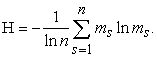(1)

If U is the universal set of the discourse, then m: U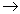[0, 1] is in formula (1) the membership function of the corresponding FS, ms = m(s) denotes the membership degree of the element s of U and n denotes the total number of the elements of U. The sum is divided by the natural logarithm of n in order to be normalized. Thus H takes values within the real interval [0, 1]. Formula (1) measures a fuzzy system’s probabilistic uncertainty.

It is recalled that the fuzzy probability of an element s of U is defined in a way analogous to the crisp probability, i.e. by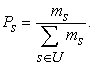(2)

However, according to Shackle  and many other researchers after him, human reasoning can be formulated more adequately by the possibility rather, than by the probability theory. The possibility, say rs , of an element s of U is defined by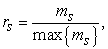(3)

where max{ms} denotes the maximal value of ms, for all s in U. In other words, the possibility of s expresses the relative membership degree of s with respect to max{ms}.

Within the domain of possibility theory uncertainty consists of strife (or discord), which expresses conflicts among the various sets of alternatives, and of non-specificity (or imprecision), which indicates that some alternatives are left unspecified, i.e. it expresses conflicts among the sizes (cardinalities) of the various sets of alternatives (, p. 28). For a better understanding of the above two types of uncertainty we give the following simple example:

EXAMPLE: Let U be the set of integers from 0 to 120 representing human ages and let Y = young, A = adult and O = old be fuzzy subsets of U defined by the membership functions mY, mA and mO respectively. People are considered as young, adult or old according to their outer appearance. Then, given x in U, there usually exists a degree of uncertainty about the values that the membership degrees mY(x), mA (x) and mO(x) could take, resulting to a conflict among the fuzzy subsets Y, A and O of U. For instance, if x = 18, values like mY(x) = 0.8 and mA (x) = 0.3 are acceptable, but they are not the only ones. In fact, the values mY(x) = 1 and mA (x) = 0.5 are also acceptable, etc. The existing conflict becomes even greater if x =50. In fact, is it reasonable in this case to take mY(x) =0? Probably not, because sometimes people being 50 years old look much younger than others aged 40 or even 30 years. But, there exist also people aged 50 who look older from others aged 70, or even 80 years! All the above are examples of the type of uncertainty that we have termed as strife.

On the other hand, non - specificity is connected to the question: How many x in U should have non zero membership degrees in Y, A and O respectively? In other words, the existing in this case uncertainty creates a conflict among the cardinalities (sizes) of the fuzzy subsets of U. It is recalled that the cardinality of a fuzzy subset, say B, of U is defined to be the sum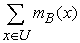of all membership degrees of the elements of U in B.-

Strife is measured (; p.28) by the function ST(r) on the ordered possibility distribution r: r1=1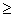r2…….rnrn+1 of a group of a system’s entities defined by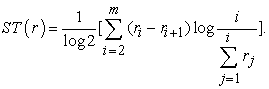(4)

Under the same conditions non-specificity is measured (; p.28) by the function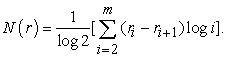(5)

The sum T(r) = ST(r) + N(r) measures the total possibilistic uncertainty for ordered possibility distributions.

### 4. Assessing the Acquisition of the van Hiele Levels by Students

Gutierrez et al.  presented a paradigm for evaluating the acquisition of the vH levels in three–dimensional Geometry by three different groups, say G1, G2 and G3, consisting of 20, 21 and 9 high school students respectively. Here, in order to illustrate the applicability of the types of a fuzzy system’s uncertainty described in Section 3 as assessment methods in the vH level theory, we shall use the data of this paradigm, which are depicted in Table 1.

#### Table 1. Data of the paradigm [Degree of acquisition]Download asPowerPoint Slide

Veiw figure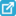View current table in a new window

In Table 1 the letters A, B, C, D and F correspond to the fuzzy linguist labels (characterizations) of excellent, very good, good, fair and non satisfactory acquisition respectively of the vH levels of geometric reasoning by students. Therefore, the set of the discourse in this paradigm is the set U = {A, B, C, D, F}.

i) Calculation of the group total possibilistic uncertainty

The data of Table 1 are characterized by a significant degree of uncertainty and fuzziness, because the student performance is not evaluated by numerical scores, but in terms of the fuzzy grades of U. Thus, although the calculation of the mean value of the scores assigned to each one of its members is the classical method for assessing the mean performance of a group of objects with respect to an action, in cases like the above the measurement of the group’s uncertainty can be used for the same purpose.

Perdikaris  used fuzzy possibilities and the corresponding fuzzy system’s possibilistic uncertainty to compare the intelligence of student groups in the vH level theory. He considered all the profiles of the form (x, y, z) with x, y and z in U representing a student’s performance in the vH levels L1, L2 and L3 respectively and he defined the membership degrees of those profiles by the product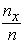.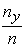.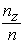of the corresponding frequencies, where the set of all profiles is represented as a fuzzy subset of U3.

However, this definition is problematic, since it assigns non – zero membership degrees to profiles like (A, B, A), (B, A, D), etc. In other words, in those profiles the student’s performance in a vH level is assumed to be worse than that in the next level, which is impossible to happen. This problem was resolved by Voskoglou in , who developed a similar model for the process of learning, by assigning non-zero membership degrees only to well defined student profiles (x, y, z). In such profiles x is a grade better or equal than y, which is better or equal than z.

The above method, although it performs a useful for the instructor/researcher quantitative analysis of all student profiles in terms of their possibilities, it is very laborious even in its revised form  requiring the calculation of the membership degrees of total student profiles - ordered samples of three taken from the five in total elements of U with replacement - and the corresponding possibilities by formulas (4) and (5) of Section 3.

Here we develop a much simpler variation of this method. For this, we represent the three student groups as fuzzy subsets of U defining their membership functions in terms of the frequencies. Under this manipulation observe that the data of the first row of Table 1, concerning the performance of G1 in level L1, imply that the ordered possibility distribution is r1=r(A)=1>r2=r3=r4=r5=0 and therefore from formulas (4) and (5) of Section 3 one finds immediately that the total possibilistic uncertainty for G1 in L1 is T1(r)= 0.

Consider now the data of the second row of Table 1 concerning the performance of G1 in level L2. In this case one finds the membership degrees m(F)=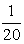, m(D)=0, m(C)=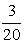, m(B)=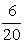and m(A)=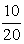. Since m(A) is the maximal membership degree, the corresponding ordered possibility distribution is r1=r(A)=1>r2= r(B)=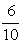>r3=r(C)=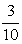>r4=r(F)=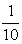>r5=r(D)=0. Therefore, since rn+1 = r5, i.e. n = 4, formula (4) gives that ST(r) =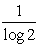[(r2-r3) log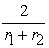+ (r3-r4) log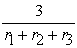+ (r4-r5) log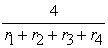] =[log (1.25) + +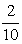log (1.58) +log 2)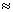0.29.

Also, formula (5) gives that N(r) =[log2+log3+log4]0.82. Therefore, the total possibilistic uncertainty for G1 in L2 is T2 (r)1.11. In the same way one finds from the third row of Table 1 that T3 (r) = 0.89 for G1 in L3{2}. Therefore, it is logical to accept that the mean value T(r) =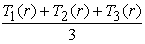0.66 measures the total possibilistic uncertainty for G1 in the first three vH levels L1, L2 and L3.

Repeating the same process for the groups G2 and G3 one finds for T(r) the values 0.85 and 1.31 respectively. Under the hypothesis that the existing uncertainty before the experiment was the same for the three groups - which practically means that the students of those groups have the same abilities{3} - this shows that G1 performed better than G2, which performed better than G3.

ii) Calculation of the group probabilistic uncertainty.

We consider again the three student groups as fuzzy subsets of U. Replacing the data of the first row of Table 1 to formula (1) of Section 3 one immediately finds that the probabilistic uncertainty for G1 in level L1 is H1 = 0. Also, replacing the membership degrees of the elements of U for G1 in L2 calculated in paragraph (i) to formula (1) one finds that H2 = -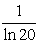(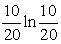+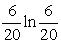+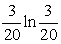+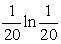)0.39. Further, for G1 in L3 one finds from the third row of Table 1 that m(A)=m(D)=, m(B)=m(C)=and m(F)=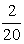. Therefore, formula (1) gives in this case that H30.51. Hence, the probabilistic uncertainty for G1 for the first three vH levels could be considered to be H =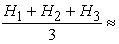0.3.

Working in the same way with the other two groups one finds the values H0.27 and H= 0.35 for G2 and G3 respectively. Therefore, under the hypothesis that the existing uncertainty before the experiment was the same for the three groups this shows that G2 performed better than G1, which performed better than G3.

### 5. Conclusion and Hints for Future Research

In this work, using the data of an experiment performed by Gutierrez et al. , we calculated the total possibilistic uncertainty and the probabilistic uncertainty for the evaluation of student group geometric reasoning in terms of the vH level theory. The calculation of the total possibilistic uncertainty, although it was performed in a way much simpler than that used in earlier works, it was proved to be laborious. On the contrary, the calculation of the probabilistic uncertainty straightforwardly from the membership degrees was much easier. However, the two methods didn’t give the same assessment outcomes. Thus, if one adopts the Shackle’s view  that the human cognition could be studied better by the possibility rather, than by the probability theory, he/she must choose the first method, although it is more laborious. Note that the measurement of the group uncertainty can be used for comparing the performance of different student groups only under the assumption that the existing for these groups uncertainty was the same before the experiment, which practically means that the students of those groups have more or less the same abilities.

Closing, we refer to our plans for future research including the calculation of the corresponding system’s uncertainty for the evaluation of several other human activities in order to obtain stronger conclusions about the method’s advantages and disadvantages. This method should also be compared with other fuzzy assessment methods, like the COG defuzzification technique [14, 21], the use of fuzzy numbers , etc.

### Notes

1. This name is due to the mathematical definition of the information I by I=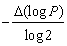, where P is the probability of appearance of each of the possible cases of the evolution of the corresponding real situation. This is analogous to the well known from Physics formula ΔS =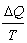, where ΔS is the increase of a physical system’s entropy caused by an increase of the heat by ΔQ, when the absolute temperature T remains constant.

2. The fact that T3 (r)< T3 (r) does not mean that G1 demonstrated a better performance in L3 than in L2, because the initially existing uncertainty was obviously different in these two levels.

3. Of course this hypothesis is not true in general.

### References

  Burger, W.P. & Shaughnessy, J.M. (1986), Characterization of the vam Hiele Levels of Development in Geometry, Journal for Research in Mathematics Education, 17, 31-48.In article View Article  Freudenthal, H. (1973), Mathematics as an Educational Task, D. Reidel, Dordrecht.In article  Fuys, D., Geddes, D. & Tischler, R. (1988), The van Hiele Model of Thinking in Geometry among Adolescents, Journal for Research in Mathematics Education, Monograph 3, NCTM, Reston, VA, USA.In article View Article  Gutierrez, A., Jaine, A. & Fortuny, J.K. (1991), An Alternative Paradigm to Evaluate the Acquisition of the van Hiele Levels, Journal for Research in Mathematics Education, 22, 237-251.In article View Article  Haviger, J. & Vozkunkova, I. (2014), The van Hiele geometry thinking levels: Gender and school type differences, Procedia – Social and Behavioral Sciences, 112, 977-981.In article View Article  Hsiu-Lan Ma et al. (2015), A study of van Hiele geometric thinking among 1st through 6th Grades, Eurasia Journal of Mathematics Science ant Technical Education, 11(5), 1181-1196.In article  Klir, G.J. & Folger, T.A. (1988), Fuzzy Sets, Uncertainty and Information, Prentice-Hall, London.In article  Klir (1995), Principles of Uncertainty: What are they? Why do we Need them? , Fuzzy Sets and Systems, 74(1), 15-31.In article  Perdikaris, S.C. (2002), Measuring the student group capacity for obtaining geometric information in the van Hiele development thought process: A fuzzy approach, Fuzzy Systems and Mathematics, 16(3), 81-86.In article  Perdikaris, S.C. (2011), Using Fuzzy Sets to Determine the Continuity of the van Hiele Levels, Journal of Mathematical Sciences and Mathematics Education, 6(3), 81-86.In article  Shackle, G.L.S. (1961), Decision, Order and Time in Human Affairs, Cambridge University Press, Cambridge, UK.In article  Shannon (1948), A mathematical theory of communications, Bell Systems Technical Journal, 27, 379-423 and 623-656In article  van Hiele, P.M. & van Hiele-Geldov, D. (1958), Report on Methods of Initiation into Geometry, edited by H. Freudenthal, J.B. Wolters, Groningen, The Netherlands, pp. 67-80.In article  van Hiele, P.M. (1986), Structure and Insight, Academic Press, New York.In article  Voskoglou, M. Gr. (1999), An Application of Fuzzy Sets to the Process of Learning, Heuristics and Didactics of Exact Sciences, 10, 9-13.In article  Voskoglou, M. Gr. (2009), Fuzziness or Probability in the Process of Learning: A General Question Illustrated by Examples from Teaching Mathematics, The Journal of Fuzzy Mathematics, International Fuzzy Mathematics Institute (Los Angeles), 17(3), 679-686.In article  Voskoglou, M. Gr. (2011), Stochastic and Fuzzy Models in Mathematics Education, Artificial Intelligence and Management, Lambert Academic Publishing, Saarbrucken, Germany.In article  Voskoglou, M. Gr. (2012), A Study on Fuzzy Systems, American Journal of Computational and Applied Mathematics, 2(5), 232-240.In article View Article  Voskoglou, M. Gr. (2013), Case-Based Reasoning in Computers and Human Cognition: A Mathematical Framework, International Journal of Machine Intelligence and Sensory Signal Processing, 1, 3-22.In article View Article  Voskoglou, M. Gr. (2015), Defuzzification of Fuzzy Numbers for Student Assessment, American Journal of Applied Mathematics and Statistics, 3(5), 206-210.In article  Voskoglou, M. Gr. (2016), Finite Markov Chain and Fuzzy Models in Management and Education, GIAN Program, Course No. 16102K03/2015-16, National Institute of Technology, Durgapur, IndiaIn article  Wilson, M. (1990), Measuring a van Hiele Geometric Sequence: A Reanalysis, Journal for Research in Mathematics Education, 21, 230-237.In article View Article